## How to Calculate and Solve for Force Settling Ratio | Fine Particles | Mineral Processing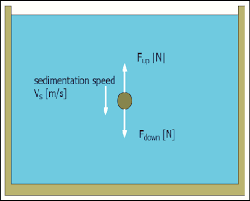The image above represents force settling ratio.

To compute for force settling ratio, three essential parameters are needed and these parameters are Density of Particle a, (ρa), Density of Particle b, (ρb) and Density of Fluid (ρf).

The formula for calculating the force settling ratio:

da/db = [ρb – ρf/ρa – ρf]0.5

Where:

da = Diameter of Particle a
db = Diameter of Particle b
ρf = Density of Fluid

Let’s solve an example;
Find the force settling ratio when the diameter of particle is 20, the diameter of particle is 16 and the density of fluid is 12.

This implies that;

da = Diameter of Particle a = 20
db = Diameter of Particle b = 16
ρf = Density of Fluid = 12

da/db = [ρb – ρf/ρa – ρf]0.5
da/db = [16 – 12/20 – 12]0.5
da/db = (4/8)0.5
da/db = (0.5)0.5
da/db = 0.707

Therefore, the force settling ratio is 0.707.

## How to Calculate and Solve for Velocity at Turbulent Flow | Mineral Processing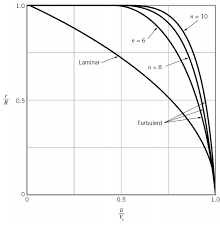The image above represents velocity at turbulent flow.

To compute for velocity at turbulent flow, four essential parameters are needed and these parameters are Acceleration due to Gravity (g), Particle Diameter (d), Density of Solid Particle (ρs) and Density of Fluid (ρf).

The formula for calculating velocity at turbulent flow:

v = [3gd(ρs – ρf)/ρf]0.5

Where:

v = Velocity at Turbulent Flow
g = Acceleration due to Gravity
d = Particle Diameter
ρs = Density of Solid Particle
ρf = Density of Fluid

Let’s solve an example;
Find the velocity at turbulent flow when the acceleration due to gravity is 14, the particle diameter is 10, the density of solid particle is 24 and the density of fluid is 12.

This implies that;

g = Acceleration due to Gravity = 14
d = Particle Diameter = 10
ρs = Density of Solid Particle = 24
ρf = Density of Fluid = 12

v = [3gd(ρs – ρf)/ρf]0.5
v = [3(14)(10)(24 – 12)/12]0.5
v = [3(14)(10)(12)/12]0.5
v = [5040/12]0.5
v = (420)0.5
v = 20.49

Therefore, the velocity at turbulent flow is 20.49 m/s.

## How to Calculate and Solve for Newton’s Drag Force for Turbulent Resistance | Mineral Processing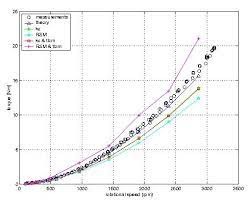The image above represents newton’s drag force for turbulent resistance.

To compute for newton’s drag force for turbulent resistance, three essential parameters are needed and these parameters are Particle Diameter (d), Terminal Velocity (v) and Density of Fluid (ρf).

The formula for calculating newton’s drag force for turbulent resistance:

D = 0.055π.d²v²ρf

Where:

D = Newton’s Drag Force for Turbulent Resistance
d = Particle Diameter
v = Terminal Velocity
ρf = Density of Fluid

Let’s solve an example;
Find the newton’s drag force for turbulent resistance when the particle diameter is 8, the terminal velocity is 4 and the density of fluid is 2.

This implies that;

d = Particle Diameter = 8
v = Terminal Velocity = 4
ρf = Density of Fluid = 2

D = 0.055π.d²v²ρf
D = 0.055π.(8)²(4)²(2)
D = 0.055π.(64)(16)(2)
D = 0.055π.(2048)
D = 353.86

Therefore, the newton’s drag force for turbulent resistance is 353.86 N.

Calculating the Particle Diameter when the Newton’s Drag Force for Turbulent Resistance, the Terminal Velocity and the Density of Fluid is Given.

d = √(D / 0.055π.v²ρf)

Where:

d = Particle Diameter
D = Newton’s Drag Force for Turbulent Resistance
v = Terminal Velocity
ρf = Density of Fluid

Let’s solve an example;
Find the particle diameter when the newton’s drag force for turbulent resistance is 42, the terminal velocity is 2 and the density of fluid is 4.

This implies that;

D = Newton’s Drag Force for Turbulent Resistance = 42
v = Terminal Velocity = 2
ρf = Density of Fluid = 4

d = √(D / 0.055π.v²ρf)
d = √(42 / 0.055π.(2²)(4))
d = √(42 / 0.055π.16)
d = √(42 / 2.76)
d = √15.2
d = 3.89

Therefore, the particle diameter is 3.89.

## How to Calculate and Solve for Velocity of Fine Particles | Mineral Processing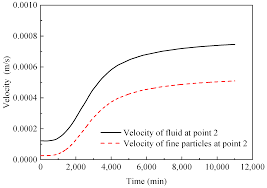The image above represents velocity of fine particles.

To compute for velocity of fine particles, four essential parameters are needed and these parameters are Constant for Fluid (k1), Particle Diameter (d), Density of Solid Particle(s) (ρs) and Density of Fluid (ρf).

The formula for calculating the velocity of fine particles:

v = k1d²(ρs – ρf)

Where:

v = Velocity of Fine Particles
k1 = Constant for Fluid
d = Particle Diameter
ρs = Density of Solid Particle(s)
ρf = Density of Fluid

Let’s solve an example;
Find the velocity of fine particles when the constant for fluid is 12, the particle diameter is 8, the density of solid particle is 4 and the density of fluid is 10.

This implies that;

k1 = Constant for Fluid = 12
d = Particle Diameter = 8
ρs = Density of Solid Particle(s) = 4
ρf = Density of Fluid = 10

v = k1d²(ρs – ρf)
v = 12(8)²(4 – 10)
v = 12(64)(-6)
v = -4608

Therefore, the velocity of fine particles is -4608 m/s.

Calculating the Constant for Fluid when the Velocity of Fine Particles, the Particle Diameter, the Density of Solid Particle and the Density of Fluid is Given.

k1 = v / d²(ρs – ρf)

Where:

k1 = Constant for Fluid
v = Velocity of Fine Particles
d = Particle Diameter
ρs = Density of Solid Particle(s)
ρf = Density of Fluid

Let’s solve an example;
Find the constant for fluid when the velocity of fine particles is 68, the particle diameter is 4, the density of solid particle is 10 and the density of fluid is 6.

This implies that;

v = Velocity of Fine Particles = 68
d = Particle Diameter = 4
ρs = Density of Solid Particle(s) = 10
ρf = Density of Fluid = 6

k1 = v / d²(ρs – ρf)
k1 = 68 / 4²(10 – 6)
k1 = 68 / 16(4)
k1 = 68 / 64
k1 = 1.06

Therefore, the constant for fluid is 1.06.

Calculating the Particle Diameter when the Velocity of Fine Particles, the Constant for Fluid, the Density of Solid Particle and the Density of Fluid is Given.

d = √(v / k1s – ρf))

Where:

d = Particle Diameter
v = Velocity of Fine Particles
k1 = Constant for Fluid
ρs = Density of Solid Particle(s)
ρf = Density of Fluid

Let’s solve an example;
Find the particle diameter when the velocity of fine particles is 88, the constant for fluid is 12, the density of solid particle is 8 and the density of fluid is 5.

This implies that;

v = Velocity of Fine Particles = 88
k1 = Constant for Fluid = 12
ρs = Density of Solid Particle(s) = 8
ρf = Density of Fluid = 5

d = √(v / k1s – ρf))
d = √(88 / 12 (8 – 5))
d = √(88 / 12 (3))
d = √(88 / 36)
d = √2.44
d = 1.56

Therefore, the particle diameter is 1.56.

## How to Calculate and Solve for Constant for Fluid | Mineral Processing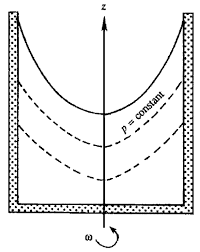The image above represents constant for fluid.

To compute for constant for fluid, two essential parameters are needed and these parameters are Acceleration due to Gravity (g) and Fluid Viscosity (μ).

The formula for calculating constant for fluid:

k1 = g / 18μ

Where:

k1 = Constant for Fluid
g = Acceleration due to Gravity
μ = Fluid Viscosity

Let’s solve an example;
Find the constant for fluid when the acceleration due to gravity is 24 and the fluid viscosity is 6.

This implies that;

g = Acceleration due to Gravity = 24
μ = Fluid Viscosity = 6

k1 = g / 18μ
k1 = 24 / 18(6)
k1 = 24 / 108
k1 = 0.22

Therefore, the constant for fluid is 0.22.

Calculating the Acceleration due to Gravity when the Constant for Fluid and the Fluid Viscosity is Given.

g = k1 x 18μ

Where:

g = Acceleration due to Gravity
k1 = Constant for Fluid
μ = Fluid Viscosity

Let’s solve an example;
Find the acceleration due to gravity when the constant for fluid is 10 and the fluid viscosity is 2.

This implies that;

k1 = Constant for Fluid = 10
μ = Fluid Viscosity = 2

g = k1 x 18μ
g = 10 x 18 (2)
g = 10 x 36
g = 360

Therefore, the acceleration due to gravity is 360.

## How to Calculate and Solve for Drag Force | Stoke’s Formula | Mineral Processing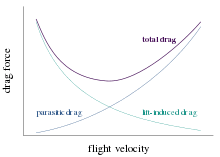The image above represents drag force.

To compute for drag force, three essential parameters are needed and these parameters are Particle Diameter (d), Fluid Viscosity (μ) and Terminal Velocity (v).

The formula for calculating drag force:

D = 3π.dμv

Where:

D = Drag Force | Stoke’s Formula
d = Particle Diameter
μ = Fluid Viscosity
v = Terminal Velocity

Let’s solve an example;
Find the drag force when the particle diameter is 21, the fluid viscosity is 7 and the terminal velocity is 3.

This implies that;

d = Particle Diameter = 21
μ = Fluid Viscosity = 7
v = Terminal Velocity = 3

D = 3π.dμv
D = 3π.(21)(7)(3)
D = 3π.(441)
D = 4156.3

Therefore, the drag force is 4156.3 N.

Calculating the Particle Diameter when the Drag Force, the Fluid Viscosity and the Terminal Velocity is Given.

d = D / 3π.μv

Where:

d = Particle Diameter
D = Drag Force | Stoke’s Formula
μ = Fluid Viscosity
v = Terminal Velocity

Let’s solve an example;
Find the particle diameter when the drag force is 30, the fluid viscosity is 4 and the terminal velocity is 2.

This implies that;

D = Drag Force | Stoke’s Formula = 30
μ = Fluid Viscosity = 4
v = Terminal Velocity = 2

d = D / 3π.μv
d = 30 / 3π.(4)(2)
d = 30 / 75.4
d = 0.39

Therefore, the particle diameter is 0.39.

## How to Calculate and Solve for Drag Force with Respect to Velocity and Density | Mineral Processing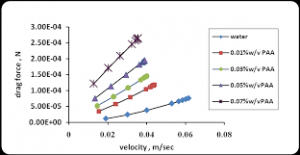The image above represents drag force with respect to velocity and density.

To compute for drag force with respect to velocity and density, four essential parameters are needed and these parameters are Particle Diameter (d), Acceleration due to Gravity (g), Density of Solid Particle (ρs) and Density of Fluid (ρf).

The formula for calculating the drag force with respect to velocity and density:

D = πd³g(ρs – ρf) / 6

Where:

D = Drag Force with Respect to Velocity and Density
d = Particle Diameter
g = Acceleration due to Gravity
ρs = Density of Solid Particle
ρf = Density of Fluid

Let’s solve an example;
Find the drag force with respect to velocity and density when the particle diameter is 6, the acceleration due to gravity is 3, the density of solid particle is 8 and density of fluid is 2.

This implies that;

d = Particle Diameter = 6
g = Acceleration due to Gravity = 3
ρs = Density of Solid Particle = 8
ρf = Density of Fluid = 2

D = πd³g(ρs – ρf) / 6
D = π(6)³(3)(8 – 2) / 6
D = π(216)(3)(6) / 6
D = 12214.5 / 6
D = 2035.75

Therefore, the drag force with respect to velocity and density is 2035.75 N.

## How to Calculate and Solve for Drag Force for Moving Particle | Mineral Processing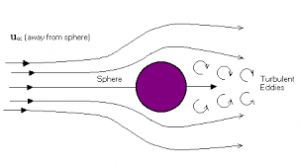The image above represents drag force for moving particle.

To compute for drag force for moving particle, three essential parameters are needed and these parameters are Mass of Solid (ms), Mass of Fluid (mf) and Acceleration due to Gravity (g).

The formula for calculating drag force for moving particle:

D = (ms – mf)g

Where:

D = Drag Force for Moving Particles
ms = Mass of Solid
mf = Mass of Fluid
g = Acceleration due to Gravity

Let’s solve an example;
Find the drag force for moving particles when the mass of solid is 14, the mass of fluid is 12 and the acceleration due to gravity is 10.

This implies that;

ms = Mass of Solid = 14
mf = Mass of Fluid = 12
g = Acceleration due to Gravity = 10

D = (ms – mf)g
D = (14 – 12)10
D = (2)10
D = 20

Therefore, the drag force for moving particles is 20 N.

Calculating the Mass of Solid when the Drag Force for Moving Particles, the Mass of Fluid and the Acceleration due to Gravity is Given.

ms = (D / g) + mf

Where:

ms = Mass of Solid
D = Drag Force for Moving Particles
mf = Mass of Fluid
g = Acceleration due to Gravity

Let’s solve an example;
Find the mass of solid when the drag force for moving particles is 10, the mass of fluid is 4 and the acceleration due to gravity is 5.

This implies that;

D = Drag Force for Moving Particles = 10
mf = Mass of Fluid = 4
g = Acceleration due to Gravity = 5

ms = (D / g) + mf
ms = (10 / 5) + 4
ms = 2 + 4
ms = 6

Therefore, the mass of solid is 6.

## How to Calculate and Solve for Sum of Forces Acting on Particle | Mineral Processing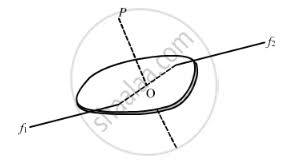The image above represents sum of forces acting on particle.

To compute for sum of forces acting on particle, two essential parameters are needed and these parameters are Mass of Particle (mp) and Acceleration (a).

The formula for calculating sum of forces acting on particle:

Σf = mp.a

Where:

Σf = Sum of Forces Acting on Particle
mp = Mass of Particle
a = Acceleration

Let’s solve an example;
Find the sum of forces acting on particle when the mass of particle is 12 and the acceleration is 4.

This implies that;

mp = Mass of Particle = 12
a = Acceleration = 4

Σf = mp.a
Σf = (12)(4)
Σf = 48

Therefore, the sum of forces acting on particle is 48 N.

## How to Calculate and Solve for Capacity of Jaw Crusher | Mineral Processing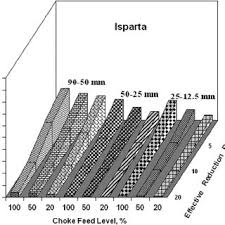The image above represents capacity of jaw crusher.

To compute for capacity of jaw crusher, three essential parameters are needed and these parameters are Gape Size (G), Width of Crusher (W) and Closed Single Setting of Jaw Crushers (CSS).

The formula for calculating capacity of jaw crusher:

CJC = G.W / CSS

Where:

CJC = Capacity of Jaw Crushers
G = Gape Size
W = Width of Crusher
CSS = Closed Single Setting of Jaw Crushers

Let’s solve an example;
Find the capacity of jaw crusher when the gape size is 10, the width of crusher is 8 and the closed single setting of jaw crusher is 14.

This implies that;

G = Gape Size = 10
W = Width of Crusher = 8
CSS = Closed Single Setting of Jaw Crushers = 14

CJC = G.W / CSS
CJC = (10)(8) / 14
CJC = (80) / 14
CJC = 5.71

Therefore, the capacity of jaw crusher is 5.71.

Calculating the Gape Size when the Capacity of Jaw Crusher, the Width of Crusher and the Closed Single Setting of Jaw Crusher is Given.

G = CJC x CSS / W

Where:

G = Gape Size
CJC = Capacity of Jaw Crushers
W = Width of Crusher
CSS = Closed Single Setting of Jaw Crushers

Let’s solve an example;
Find the gape size when the capacity of jaw crushers is 5, the width of crusher is 3 and the closed single setting of jaw crusher is 6.

This implies that;

CJC = Capacity of Jaw Crushers = 5
W = Width of Crusher = 3
CSS = Closed Single Setting of Jaw Crushers = 6

G = CJC x CSS / W
G = 5 x 6 / 3
G = 30 / 3
G = 10

Therefore, the gape size is 10.